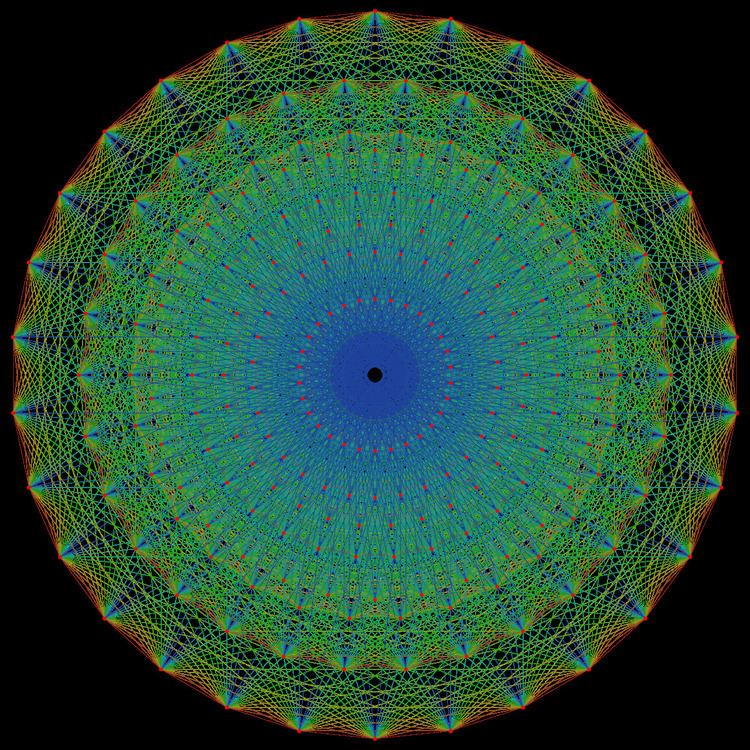# E8 polytope

Updated onIn 8-dimensional geometry, there are 255 uniform polytopes with E8 symmetry. The three simplest forms are the 421, 241, and 142 polytopes, composed of 240, 2160 and 17280 vertices respectively.

These polytopes can be visualized as symmetric orthographic projections in Coxeter planes of the E8 Coxeter group, and other subgroups.

## Graphs

Symmetric orthographic projections of these 255 polytopes can be made in the E8, E7, E6, D7, D6, D5, D4, D3, A7, A5 Coxeter planes. Ak has [k+1] symmetry, Dk has [2(k-1)] symmetry, and E6, E7, E8 have , ,  symmetry respectively. In addition there are two other degrees of fundamental invariants, order  and  for the E8 group that represent Coxeter planes.

11 of these 255 polytopes are each shown in 14 symmetry planes, with vertices and edges drawn, and vertices colored by the number of overlapping vertices in each projective position.

Similar Topics